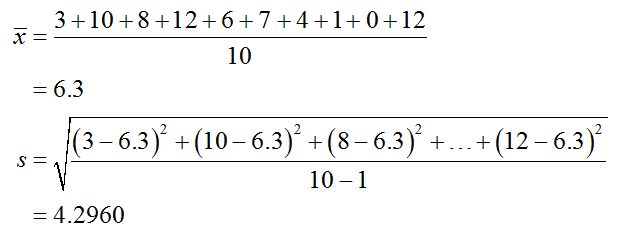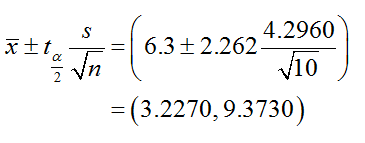# A psychiatrist is interested in finding a 95% confidence interval for the tics per hour exhibited by children with Tourette syndrome. The data below show the tics in an observed hour for 10 randomly selected children with Tourette syndrome. Round answers to 3 decimal places where possible.3108126741012a. To compute the confidence interval use a (z or t) distribution?b. With 95% confidence the population mean number of tics per hour that children with Tourette syndrome exhibit is between  and  .c. If many groups of 10 randomly selected  children with Tourette syndrome are observed, then a different confidence interval would be produced from each group. About  percent of these confidence intervals will contain the true population mean number of tics per hour and about  percent will not contain the true population mean number of tics per hour.

Question
14 views

A psychiatrist is interested in finding a 95% confidence interval for the tics per hour exhibited by children with Tourette syndrome. The data below show the tics in an observed hour for 10 randomly selected children with Tourette syndrome. Round answers to 3 decimal places where possible.

 3 10 8 12 6 7 4 1 0 12

a. To compute the confidence interval use a (z or t) distribution?

b. With 95% confidence the population mean number of tics per hour that children with Tourette syndrome exhibit is between  and  .

c. If many groups of 10 randomly selected  children with Tourette syndrome are observed, then a different confidence interval would be produced from each group. About  percent of these confidence intervals will contain the true population mean number of tics per hour and about  percent will not contain the true population mean number of tics per hour.

check_circle

Step 1

a. Distribution:

Since population standard deviation is not known and sample size is less than 30, t distribution can be used to compute the confidence interval.

Step 2

b. Computation of 95% confidence interval for the mean number of tics per hour that children with Tourette syndrome:

The sample mean and sample standard deviation is calculated as follows:The degrees of freedom is 9 (=10–1).

From t-distribution table, the critical value corresponding to t0.025 (=tα/2) and 9 df is 2.262.

The confidence interval is obtained as follows:Therefore, the 95% confidence interval for the population mean number of tics per hour that children with Tourette syndrome exhibit is between 3.2270 and 9.3730 tics....

### Want to see the full answer?

See Solution

#### Want to see this answer and more?

Solutions are written by subject experts who are available 24/7. Questions are typically answered within 1 hour.*

See Solution
*Response times may vary by subject and question.
Tagged in